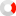Institute of Certified Bookkeepers
 First Previous - Page 1 of 1 - Next Last Oldest to newest Newest to oldest

## Diminishing Value Depreciation Formula

 Student1 post # 119149 Sat 17 Oct 2020 05:47 AM Hi everyone.I am currently undergoing studies in Bookkeeping with TAFE and was wondering if anyone was available to assist me with a formula to work with on the following activity? This isn't a graded test btw, just an activity but I would like to understand it and my lecturer is away at the moment. We were provided with a few formula but I am having trouble rearranging any to get the value I need. The question is as follows... The furniture in an office was bought 6 years ago. It is being depreciated over 10 years, straight line, to a scrap value of \$200. If its current book value is \$1,120, how much did it cost? Can anyone help derive a formula where cost = ... Thanks in advance and sorry if this is the wrong place to post.   Edit: This is straightline depreciation. Can't change title.Edited at 17 Oct 2020 06:57 AM GMT 15 posts # 119174 Thu 22 Oct 2020 01:12 PM Diminishing value depreciationDiminishing value depreciation reduces the book value of an asset at a higher rate in the earlier years it's owned. Xero calculates: Annual depreciation by multiplying the depreciable value of the asset less previous depreciation, by the depreciation rateMonthly depreciation using the actual days averaging methodFor example, if the depreciable value of an asset is 1300 and you: Enter a rate of 20%, the annual depreciation for the first three years is: First year – 260 (1300 x 20% = 260) Second year – 208 (1300 - 260 = 1040), (1040 x 20% = 208) Third year – 166.40 (1040 - 208 = 832), (832 x 20% = 166.40) Enter an effective life of 8 years with Diminishing Value (150%), the rate is 18.75%, and the annual depreciation for the first three years is: First year – 243.75 (150% ÷ 8 = 18.75%), (1300 x 18.75% = 243.75) Second year – 198.05 (1300-243.75 = 1056.25), (1056.25 x 18.75% = 198.05) Third year – 160.91 (1056.25 - 198.05 = 858.20) (858.20 x 18.75% = 160.91)
 First Previous - Page 1 of 1 - Next Last Oldest to newest Newest to oldest